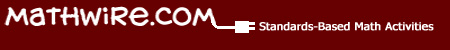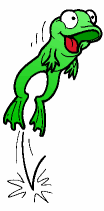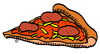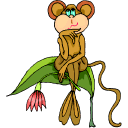# Fractions

## Problem Solving

Students are challenged to solve these problems by drawing pictures rather than using traditional fraction algorithms.•Animal Shelter requires students to use fractions to figure out how many cats and dogs were available for adoption at the animal shelter.
•Tadpoles and Frogs requires students to use fractions to figure out how many tadpoles and frogs there were in the tank.
•Fraction Game simulates a Fraction War game but students must draw a representation of each fraction and explain who won, based on the drawings.
•High Number Game requires that students apply their knowledge of fractions, decimals and percents to decide whose number card is highest.
•Pattern Block Fraction Design requires students to use pattern blocks to fill the shape so that it has a line of symmetry. They must then write a fraction for each color pattern block used in the design.•The Adventures of Mrs. Meatball problem set challenges students to draw pictures to solve each problem and prove their answers.
• Use theFraction Circles Template for students to divide and color the fractional pieces, if desired.•Monkey Business challenges students to work backwards to solve this fraction division problem.
•Bake Sale challenges students to work backwards to solve another fraction division problem involving disappearing cookies.